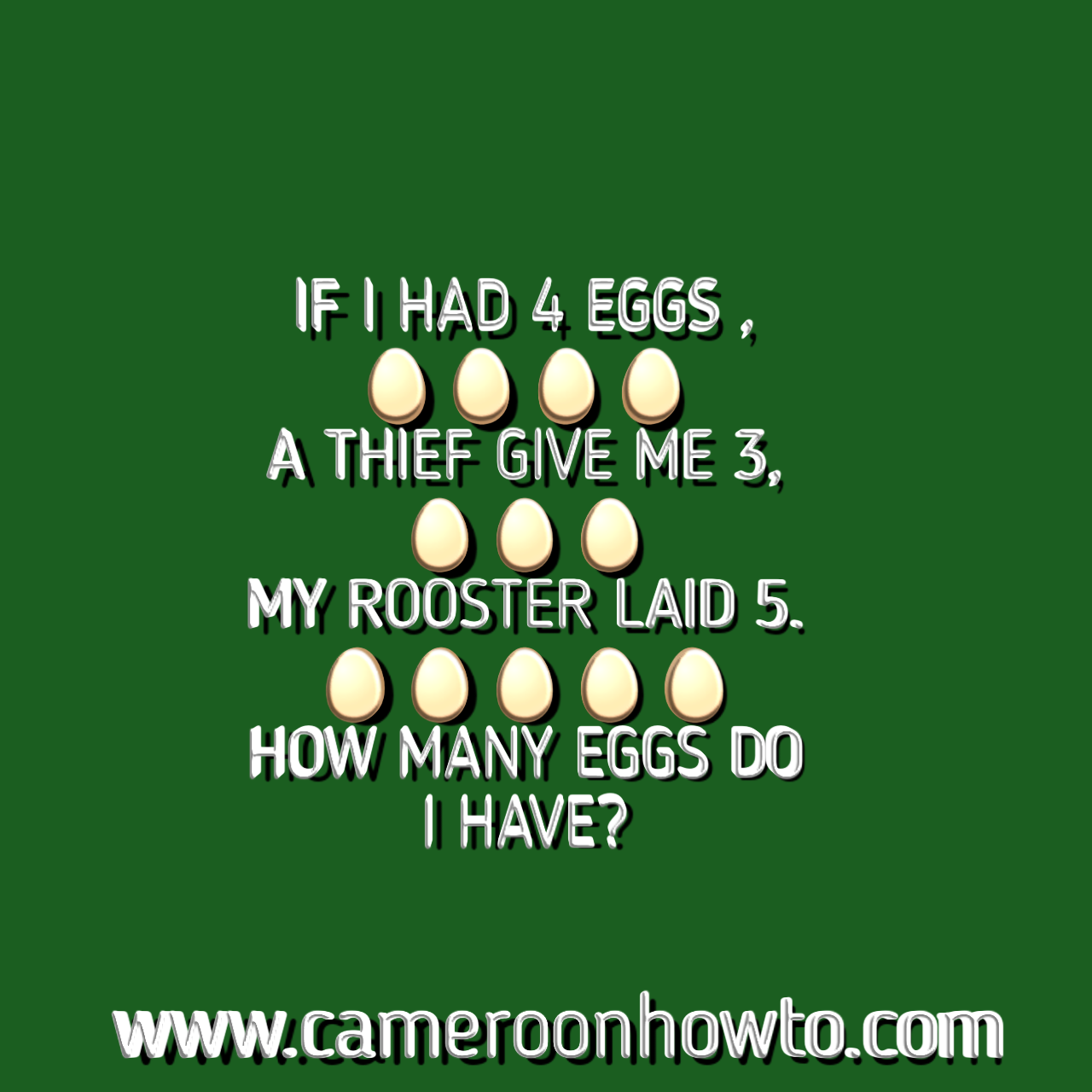0If I had four eggs Puzzle answer
This picture and common sense math Puzzle is one of those trending on social media today. Many people are sharing different views and answers. Have you seen the " If I had four eggs riddle before?" If this is your first time seeing it, try to solve and check my own well explained answer below.

The puzzle was posted on Facebook and many people failed it. Be sure to share with your friends and family members to test them too. The puzzle is If I had four eggs ,

a thief gave me 3,

my rooster laid 5.

How many eggs do I have?"

## If I had four eggs, how many eggs do I have picture Puzzle answer

Mathematically, the correct answer would be 12 not 0, 3 or 7  because many Math riddles and Puzzles are based on assumptions, there's also a common sense part in the puzzle that says the answer is 3 or 0. check details below.

I will answer the Puzzle mathematically and also explain the common sense part in it then concludes

Simple way to answer is to sum up all the eggs.

Let's sum: 4 eggs + 3 eggs + 5eggs  = 12 eggs.

So, the math answer is 12.

If I had four eggs. It means I didn't have so eggs= Zero

A thief gave me three: so I have three eggs. But in other common sense, I can't take eggs from a thief to implicate myself. Would you? If Yes then, your answer here is 3 if no then it's Zero

My rooster laid five: This is wrong because Roosters don't lay eggs. So eggs = Zero.

Therefore the common sense answer is Zero if you can't receive eggs from a thief or 3 if you can receive eggs from a thief.

### Conclusion

The best and correct answer to "How many eggs do I have?" Is 12. This is because math puzzles and riddles are always based on assumptions. Only a few will be based on common sense.

If you want to apply common sense, many answers will always be Zero which doesn't make sense mathematically.

That's why "if" is usually used when setting a question because some of these Puzzles are just to catch fun and relax oneself.

Also check Answer to:How many dolphins can you see math picture Puzzle